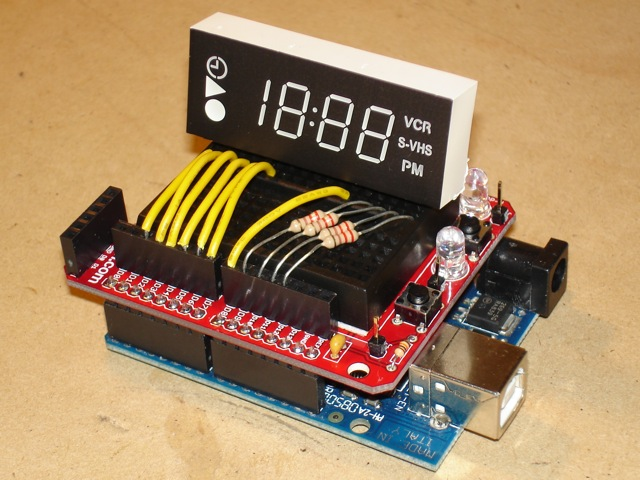Select Page

Difficulty Level = 3 [What’s this?]

I harvested this LED clock display from an ancient VCR and wrote an Arduino sketch to drive the display. First I experimented with the leads to understand which leads controlled what behavior. There are 4 digits on the display and there are 4 corresponding leads for positive voltage to drive them (the Arduino pins connected with the resistors). Then there are 7 ground pins (the yellow wires) that correspond to the seven segments on each digit (well, the leftmost digit only has 2 segments). There’s also a pin for the colon but one of the LEDs is burned out.ClockShield!

This display is driven in a typical manner by multiplexing between the 7 segments of each digit. The display is driven by sinking current on each of the seven segments in sequence, and sending current into the leads for any digit that needs to display that segment. Ouch, that’s confusing, so I’ll give an example. The first display ground pin (connected to pin 2 on the Arduino) is for the horizontal middle segment. Some numbers need the horizontal middle segment turned on, right? Numbers ‘0’, ‘2’, ‘3’, ‘4’, ‘5’, ‘6’, ‘8’, and ‘9’. So, the code sets the ground pin for this segment to LOW (to sink current), and for each of the four digits that need that segment, we set the digit pin to HIGH. If the number to display is ‘1027’, then when Arduino pin 2 is LOW (for the horizontal middle segment), we set the digit pins HIGH for the two middle digits (the ‘0’ and the ‘2’). Then we repeat this process for each of the 7 segments. If we do it fast enough, then persistence of vision makes it look like the LEDs are all on at the same time.

If you are unfamiliar with this multiplexing I’ve tried to explain, then study the code for the sketch. The array ‘number’ contains the information about what segments need to be on for each number. This might seem hard to understand at first, but I can guarantee an “ah ha!” moment when you get it.

```// These are indexes into the groundPins array
#define MIDDLE 0
#define UPPER_L 1
#define LOWER_L 2
#define BOTTOM 3
#define LOWER_R 4
#define UPPER_R 5
#define TOP 6

int groundPins = {2, 3, 4, 5, 6, 7, 8};
int digitPins = {9, 10, 11, 12}; // positive voltage supply for each of the 4 digits
int ON = HIGH;
int OFF = LOW;
int number; // holds information about which segments are needed for each of the 10 numbers
int digit; // holds values for each of the 4 display digits

int hours = 12;
int minutes = 8;
int elapsedMinutes = 0;
int seconds = 0;

void setup()
{
initNumber();

for(int i=0; i < 7; i++) {
pinMode(groundPins[i], OUTPUT);
digitalWrite(groundPins[i], HIGH);
}
for(int i=0; i < 4; i++) {
pinMode(digitPins[i], OUTPUT);
digitalWrite(digitPins[i], LOW);
}
}

void loop() {
int n = 0;
unsigned long time = millis() - (elapsedMinutes * 60000);
seconds = (time / 1000);
if (seconds > 60) {
seconds = 0;
minutes++;
elapsedMinutes++;
if (minutes >= 60) {
minutes = 0;
hours++;
if (hours > 12) {
hours = 1;
}
}
}

n = (hours * 100) + minutes;
setDigit(n);

for(int g=0; g < 7; g++) {
digitalWrite(groundPins[g], LOW);
for(int i=0; i < 4; i++) {
if (digit[i] < 0) {
continue;
}
digitalWrite(digitPins[i], number[digit[i]][g]);
}
delay(getDelay());
digitalWrite(groundPins[g], HIGH);
}
}

void setDigit(int n) {
n = n % 2000;
digit = n % 10;
digit = (n / 10) % 10;
if ((digit == 0) && (n < 10)) {
digit = -1;
}
digit = (n / 100) % 10;
if ((digit == 0) && (n < 100)) {
digit = -1;
}
digit = (n / 1000) % 10;
if (digit == 0) {
digit = -1;
}
}

int getDelay() {
if (millis() > 10000) {
return 0;
} else {
return (int) (((10000 - millis()) / 10000.0) * 125);
}
}

void initNumber() {
number[MIDDLE]  = OFF;
number[UPPER_L] = ON;
number[LOWER_L] = ON;
number[BOTTOM]  = ON;
number[LOWER_R] = ON;
number[UPPER_R] = ON;
number[TOP]     = ON;

number[MIDDLE]  = OFF;
number[UPPER_L] = OFF;
number[LOWER_L] = OFF;
number[BOTTOM]  = OFF;
number[LOWER_R] = ON;
number[UPPER_R] = ON;
number[TOP]     = OFF;

number[MIDDLE]  = ON;
number[UPPER_L] = OFF;
number[LOWER_L] = ON;
number[BOTTOM]  = ON;
number[LOWER_R] = OFF;
number[UPPER_R] = ON;
number[TOP]     = ON;

number[MIDDLE]  = ON;
number[UPPER_L] = OFF;
number[LOWER_L] = OFF;
number[BOTTOM]  = ON;
number[LOWER_R] = ON;
number[UPPER_R] = ON;
number[TOP]     = ON;

number[MIDDLE]  = ON;
number[UPPER_L] = ON;
number[LOWER_L] = OFF;
number[BOTTOM]  = OFF;
number[LOWER_R] = ON;
number[UPPER_R] = ON;
number[TOP]     = OFF;

number[MIDDLE]  = ON;
number[UPPER_L] = ON;
number[LOWER_L] = OFF;
number[BOTTOM]  = ON;
number[LOWER_R] = ON;
number[UPPER_R] = OFF;
number[TOP]     = ON;

number[MIDDLE]  = ON;
number[UPPER_L] = ON;
number[LOWER_L] = ON;
number[BOTTOM]  = ON;
number[LOWER_R] = ON;
number[UPPER_R] = OFF;
number[TOP]     = ON;

number[MIDDLE]  = OFF;
number[UPPER_L] = OFF;
number[LOWER_L] = OFF;
number[BOTTOM]  = OFF;
number[LOWER_R] = ON;
number[UPPER_R] = ON;
number[TOP]     = ON;

number[MIDDLE]  = ON;
number[UPPER_L] = ON;
number[LOWER_L] = ON;
number[BOTTOM]  = ON;
number[LOWER_R] = ON;
number[UPPER_R] = ON;
number[TOP]     = ON;

number[MIDDLE]  = ON;
number[UPPER_L] = ON;
number[LOWER_L] = OFF;
number[BOTTOM]  = ON;
number[LOWER_R] = ON;
number[UPPER_R] = ON;
number[TOP]     = ON;
}```

Now for the fun. The getDelay() method returns a delay based on how long the sketch has been running. First we start with a longer delay (125ms) between each segment so you can see visually how the digits are being rendered. Over the course of 10 seconds, we reduce the delay to 0. This way you can see exactly how quickly rendering the display causes you to see the digits without ficker even though each segment is being displayed separately! Cool, huh?